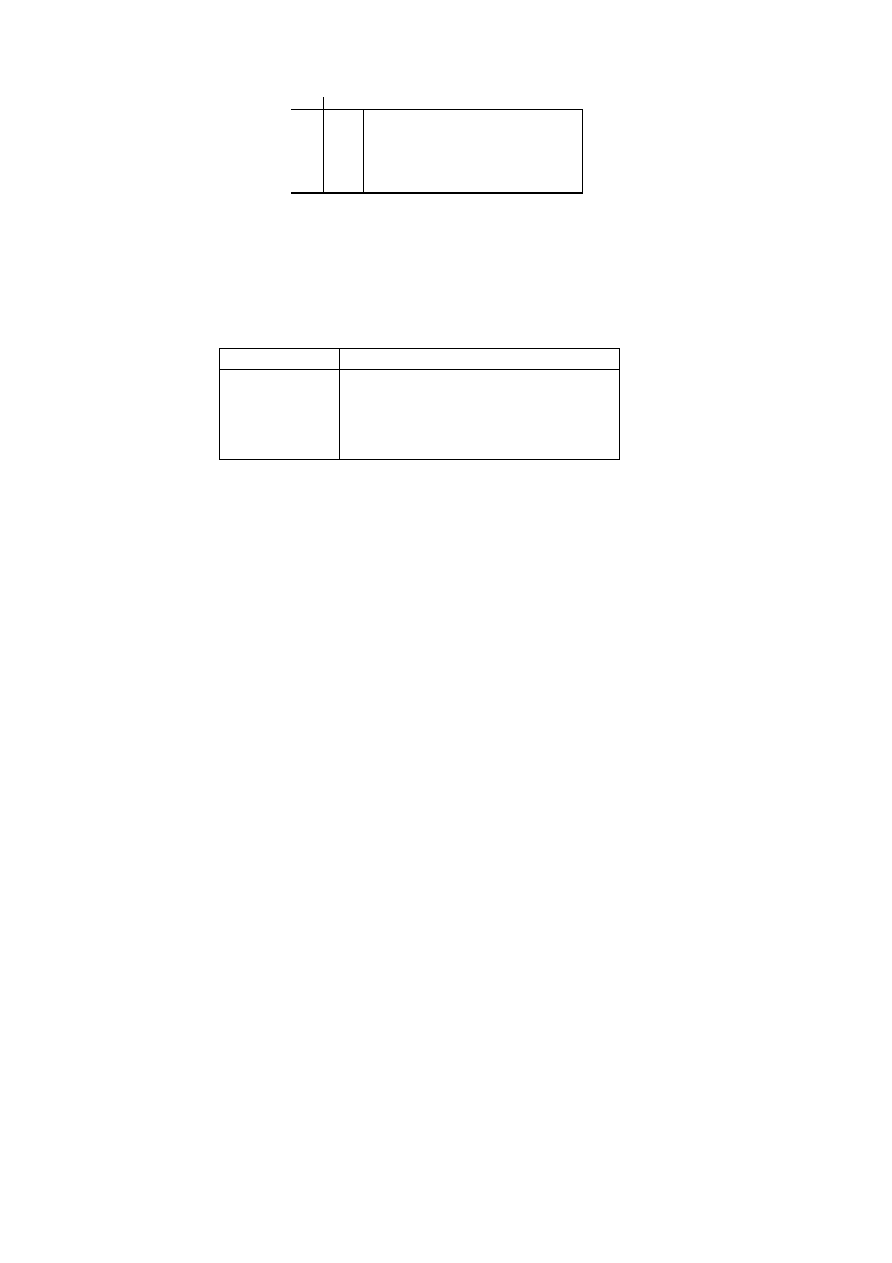ISO/IEC 10918-1 : 1993(E)
0
1
2
0
.
.
.
15
EOB
N/A
N/A
N/A
ZRL
. . .
RRRR
SSSS
TISO1430-93/d081
13
14
COMPOSITE VALUES
Figure F.11 ­ Two-dimensional value array for Huffman coding
Figure F.11 {D81] = 4.5 cm = 176 %
Table F.7 ­ Values assigned to coefficient amplitude ranges
SSSS
AC coefficients
11
­2 047..­1 024,1 024..2 047
12
­4 095..­2 048,2 048..4 095
13
­8 191..­4 096,4 096..8 191
14
­16 383..­8 192,8 192..16 383
F.1.6
Extended sequential DCT-based arithmetic encoding process for 12-bit sample precision
The process is identical to the sequential DCT process for 8-bit precision except for changes in the precision of the FDCT
computation.
The structure of the encoding procedure is identical to that specified in F.1.4 which was already defined for a 12-bit
sample precision.
F.2
Sequential DCT-based decoding processes
F.2.1
Sequential DCT-based control procedures and coding models
F.2.1.1
Control procedures for sequential DCT-based decoders
The control procedures for decoding compressed image data and its constituent parts ­ the frame, scan, restart interval and
MCU ­ are given in Figures E.6 to E.10. The procedure for decoding a MCU (Figure E.10) repetitively calls the
procedure for decoding a data unit. For DCT-based decoders the data unit is an 8
×
8 block of samples.
F.2.1.2
Procedure for decoding an 8
×
×
8 block data unit
In the sequential DCT-based decoding process, decoding an 8
×
8 block data unit consists of the following procedures:
a)
decode DC coefficient for 8
×
8 block using the DC table destination specified in the scan header;
b)
decode AC coefficients for 8
×
8 block using the AC table destination specified in the scan header;
c)
dequantize using table destination specified in the frame header and calculate the inverse 8
×
8 DCT.
F.2.1.3
Decoding models for the sequential DCT procedures
Two decoding procedures are used, one for the DC coefficient ZZ(0) and the other for the AC coefficients ZZ(1)...ZZ(63).
The coefficients are decoded in the order in which they occur in the zig-zag sequence order, starting with the DC
coefficient. The coefficients are represented as two's complement integers.
CCITT Rec. T.81 (1992 E)
103